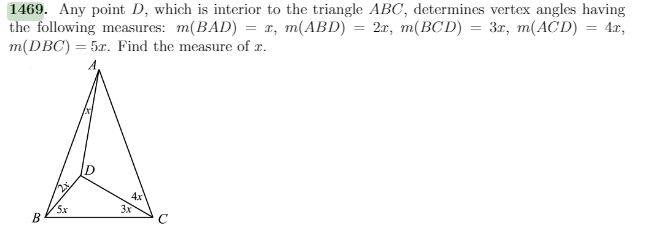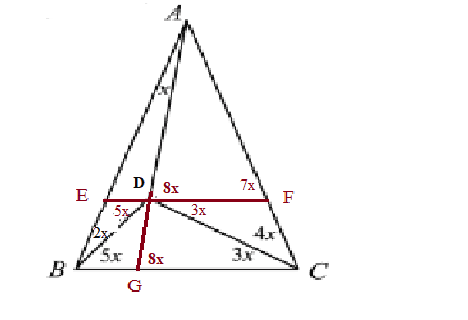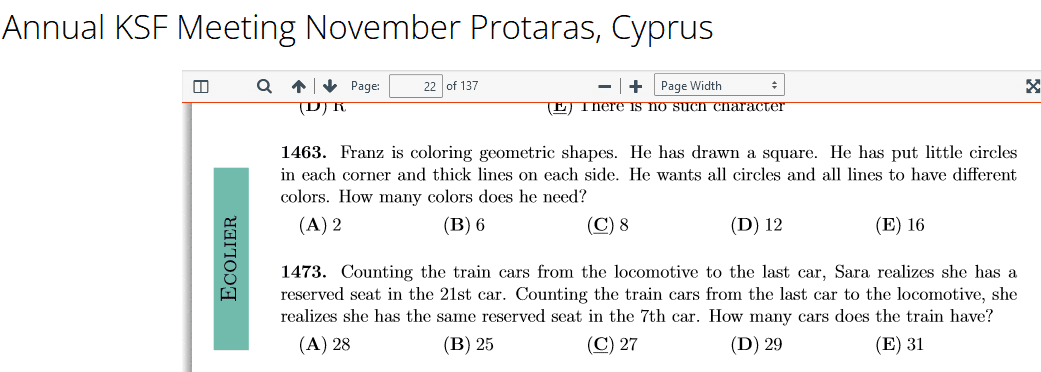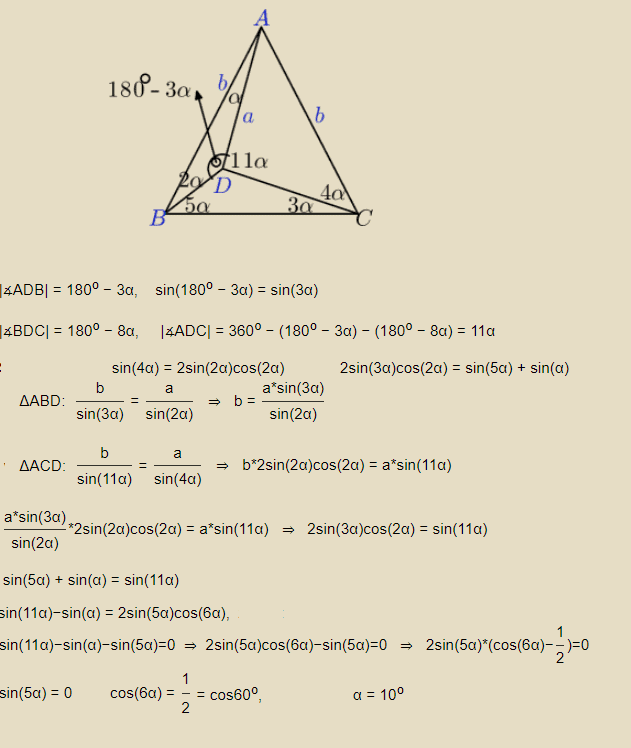# Find the angle in the triangle

• MHB
maxkorI've tried:
BC || EF
How to find angle GDC? I think GDC=7x but why?
I have an answer but how to solve this?

Last edited:

Gold Member
MHB
https://www.physicsforums.com/attachments/11871
View attachment 11873

I've tried:
BC || EF
How to find angle GDC? I think GDC=7x but why?
I have an answer but how to solve this?
There's another approach. Look at angle BAC. It's 180 - 7x - 7x. (ABC is isosceles, but we don't need this.) So we can get angle DAC in terms of x.

Now find the angles ADB, BDC, and ADC. They sum to 180...

-Dan

maxkor
BAC=180-15x
BDC=180-8x
What next?

Last edited:
Gold Member
MHB
Check that again. BAC = 180 - 14x.

-Dan

maxkor
ok, BAC = 180 - 14x
Can you tel why ADB + BDC + ADC= 180? I don't see it.

Gold Member
MHB
ok, BAC = 180 - 14x
Can you tel why ADB + BDC + ADC= 180? I don't see it.
Sorry, that was a typo. They sum to 360. Notice that all three angles sum to form a circle.

-Dan

maxkor
BDC=180-8x
And what next??

Gold Member
MHB
BDC=180-8x
And what next??
Well, it made a lot more sense when I had the typo! :)

I'll have to get back to you on this. Sorry about that!

-Dan

Homework Helper
MHB
Picture to scale with $x=0.5^\circ$ where the angles are correct except for $\angle ACD$.
\begin{tikzpicture}
\def\x{0.5}
\def\d{4}
\def\b{\d * sin(3*\x) / sin(2*\x)}
\def\a{(\b) * sin(14*\x) / sin(7*\x)}
\def\e{(\b) * sin(\x) / sin(3 * \x)}
\coordinate[label=left:B] (B) at (0,0);
\coordinate[label=A] (A) at ({7*(\x)}:{\b});
\coordinate[label=D] (D) at ({5*\x}:{\e});
\coordinate[label=right:C] (C) at ({\a},0);
\draw (A) -- (B) -- (C) -- cycle (B) -- (D) -- (C) (D) -- (A);
\end{tikzpicture}
Note that $\angle ACD=4x$ is too big in the picture.
Btw, this is TikZ code in which we can change the angle $x$ if we want to play with it.

Solution

The angles at B and C are both $7x$. Therefore the triangle is isosceles, and
$$AB=AC$$

From the law of sines:
$$\triangle ABD:\quad\frac{AB}{\sin(180^\circ-3x)}=\frac{AD}{\sin(2x)}$$
$$\triangle ACD:\quad\frac{AC}{\sin(11x)}=\frac{AD}{\sin(4x)}$$

Combine to find:
$$\frac{AB}{AD}=\frac{\sin(3x)}{\sin(2x)}=\frac{\sin(11x)}{\sin(4x)} \implies \sin(3x)\sin(4x)=\sin(2x)\sin(11x)\tag 4$$

Wolfram seems to say that $x=0$.

EDIT: with some twiddling, $x=10^\circ$ rolls out as the only solution in which we actually have a triangle.

Last edited:
Homework Helper
MHB
Ok but answer is 10 not 0, so how solve this without wolfram.
I've tried to find a trick solution.
Interesting, Wolfram confirms that $x=10^\circ$ is also a solution of the same equation. It appears that I misinterpreted the output of Wolfram.

\begin{tikzpicture}
\def\x{10}
\def\d{4}
\def\b{\d * sin(3*\x) / sin(2*\x)}
\def\a{(\b) * sin(14*\x) / sin(7*\x)}
\def\e{(\b) * sin(\x) / sin(3 * \x)}
\coordinate[label=left:B] (B) at (0,0);
\coordinate[label=A] (A) at ({7*(\x)}:{\b});
\coordinate[label=D] (D) at ({5*\x}:{\e});
\coordinate[label=right:C] (C) at ({\a},0);
\draw (A) -- (B) -- (C) -- cycle (B) -- (D) -- (C) (D) -- (A);
\end{tikzpicture}

Last edited:
maxkor
But that equation is hard to solve.

maxkor
This is the problem with the 4 point kangaroo competition.

Homework Helper
MHB
This is the problem with the 4 point kangaroo competition.
There doesn't seem to be a problem 1469 in there:Homework Helper
MHB
I've tried to find a trick solution.

If only we could prove that $\triangle CGD$ is similar to $\triangle AGC$. 🤔
We have 1 angle that is the same.
We either need another angle, or we need to prove $\triangle CGD$ is also isosceles.

Last edited:
maxkor
Look page 74.

maxkor
If only we could prove that $\triangle CGD$ is similar to $\triangle AGC$. 🤔
We have 1 angle that is the same.
We either need another angle, or we need to prove $\triangle CGD$ is also isosceles.
$\triangle CGD$ is not also isosceles.
If only we could prove that $\triangle CGD$ is similar to $\triangle AGC$, if GDC=7x ??

Homework Helper
MHB
Turns out that there are $13$ integer solutions for $x$ in degrees between $0^\circ$ and $180^\circ$. And those are the only solutions.
The solution $x=10^\circ$ is the only one such that all angles of the triangle are less than $180^\circ$.

The interesting part is that angles with an integer number of degrees are solutions.
For that to make sense, there must be angles hidden somewhere of a multiple of $x$ that is $90^\circ$ so that the cosine is zero, or $180^\circ$ so that the sine is zero.

Put otherwise, if we can find an angle of $9x$ somewhere (or $18x$), and if we can prove that it is a right angle (respectively straight angle), then the solution $x=10^\circ$ comes rolling out.
Unfortunately I haven't found an angle of $9x$ yet, although I do have $x,2x,3x,4x,5x,7x,8x,10x,11x,12x$ and their complements.

maxkor
Maybe you have GDC=7x? we at home :)

maxkor